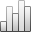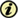Acta Stereologica

0351-580XPlus de Statistiquesya que 05 diciembre 2013 :
Vista(s): 399 (3 ULiège)
Descargar(s): 45 (1 ULiège)
Stephen L. Gay

### A geometric probability approach to estimating particle volume distribution

(Volume 15 (1996) — Number 1 - Mathematical foundations of stereology - Feb. 1996)
Article#### Abstract

A fundamental problem in stereology is to estimate the particle volume distribution using planar proﬁles. A model has been developed to solve this problem. The model is based on three geometric probability equations and a small number of assumptions. These assumptions are that sectioning is isotropic and uniform, particle shape changes smoothly with particle volume and particles are convex. The model is based on dividing the particle volume distribution into bins with each bin representing a narrow range in particle volume. The planar proﬁles are allocated into the bins. The method of allocation is to ensure that for each bin the volume estimates obtained from applying the three geometric probability equations correspond closely to the bin particle volume. The model is verified by numerical simulation and experiment. The numerical simulation is based on sectioning ellipsoids. For the simulations the model for estimating particle volume distribution from planar proﬁles was found to be very accurate. The experimental verification has the advantage of testing the model on 'real' particles. The particle volume distribution model was applied to planar proﬁles from particles with a known size distribution. For this verification the estimated particle volume distribution was not as accurate as for the numerical simulations. The reason for this is most likely experimental error.

Keywords : stereology, geometric probability, integral geometry, particle volume distribution, size distribution

#### Para citar este artículo

Stephen L. Gay, «A geometric probability approach to estimating particle volume distribution», Acta Stereologica [En ligne], Volume 15 (1996), Number 1 - Mathematical foundations of stereology - Feb. 1996, 25-30 URL : https://popups.ulg.ac.be/0351-580x/index.php?id=409.

#### Acerca de: Stephen L. Gay

Julius Kruttsclmitt Mineral Research Centre, Isles Rd., Indooroopilly, 4068, Australia